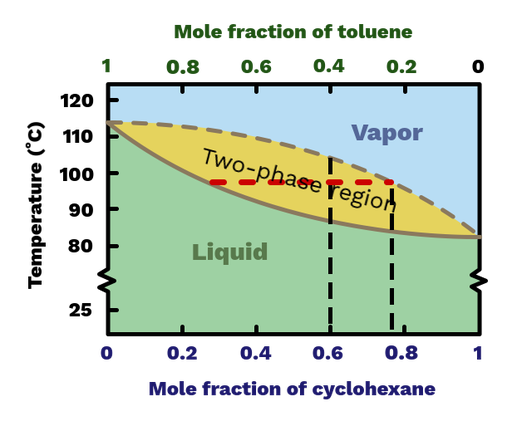Boiling point vs. composition diagrams is an effective tool for understanding distillation, and you can see the one for cyclohexane and toluene mixtures in Figure 1.

At the left and right sides of the diagram, we find the pure compounds, in this case, cyclohexane and toluene. Between these two sides, we have a mix of the two components. The x-axis, therefore, shows the mole fraction or mol% of the two components. The y-axis shows the temperature in °C. At a given temperature, the two curves in the diagram tell you the mole percent of the liquid (solid line) and vapor (dotted line). Between these two lines, we have a two-phase region. This region appears due to mixing interactions between the two components.Figure 1: Boiling point vs. composition diagram for mixtures of cyclohexane and toluene.

Let's say you heated at 50:50 mixture of cyclohexane and toluene (i.e., 50 mol% of both) to 100 °C. In this case, we would be in the two-phase region between the two lines, meaning that we have a mixture of liquid and vapor. By drawing a horizontal line across the diagram at 100 °C, we see that the liquid and vapor contain ca. 20 mol% and 80 mol% cyclohexane, respectively.